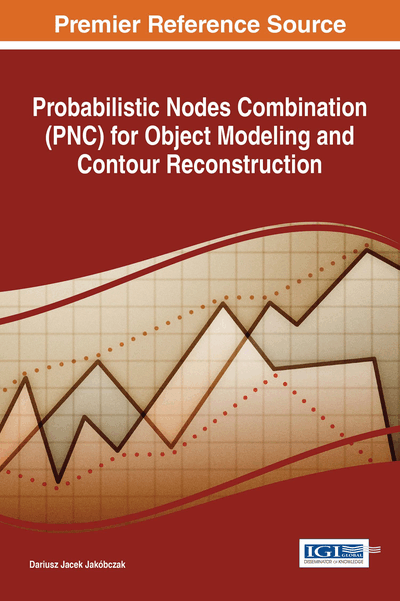# Applications of PNC in Artificial Intelligence

DOI: 10.4018/978-1-5225-2531-8.ch008
Available
\$37.50
No Current Special Offers

## Abstract

Interpolation methods and curve fitting represent so huge problem that each individual interpolation is exceptional and requires specific solutions. Presented method is such a new possibility for curve fitting and interpolation when specific data (for example handwritten symbol or character) starts up with no rules for polynomial interpolation. The method of Probabilistic Nodes Combination (PNC) enables interpolation and modeling of two-dimensional curves using nodes combinations and different coefficients ?. This probabilistic view is novel approach a problem of modeling and interpolation. Computer vision and pattern recognition are interested in appropriate methods of shape representation and curve modeling. PNC method represents the possibilities of shape reconstruction and curve interpolation via the choice of nodes combination and probability distribution function for interpolated points. It seems to be quite new look at the problem of contour representation and curve modeling in artificial intelligence and computer vision.
Chapter Preview
Top

## Introduction

Probabilistic modeling is still developing branch of the computer science: operational research (for example probabilistic model-based prognosis) (Lorton, Fouladirad & Grall, 2013), decision making techniques and probabilistic modeling (Pergler & Freeman, 2008), artificial intelligence and machine learning. There are used different aspects of probabilistic methods: stochastic processes and stochastic model-based techniques, Markov processes (Cocozza-Thivent, Eymard, Mercier & Roussignol, 2006), Poisson processes, Gamma processes, a Monte Carlo method, Bayes rule, conditional probability and many probability distributions. In this chapter the goal of probability distribution function is to describe the position of unknown points between given interpolation nodes. Two-dimensional curve (opened or closed) is used to represent the data points. So problem statement of this chapter is: how to reconstruct (interpolate) missing points of 2D curve having the set of interpolation nodes (key points) and using the information about probabilistic distribution of unknown points. For example the simplest basic distribution leads to the easiest interpolation – linear interpolation. Apart from probability distribution, additionally there is the second factor of proposed interpolation method: nodes combination. The simplest nodes combination is zero. Thus proposed curve modeling is based on two agents: probability distribution and nodes combination. Curve interpolation (Collins, 2003) represents one of the most important problems in mathematics and computer science: how to model the curve (Chapra, 2012) via discrete set of two-dimensional points (Ralston & Rabinowitz, 2001)? Also the matter of shape representation (as closed curve-contour) and curve parameterization is still opened (Zhang & Lu, 2004). For example pattern recognition, signature verification or handwriting identification problems are based on curve modeling via the choice of key points. So interpolation is not only a pure mathematical problem but important task in computer vision and artificial intelligence. The chapter wants to approach a problem of curve modeling by characteristic points. Proposed method relies on nodes combination and functional modeling of curve points situated between the basic set of key points. The functions that are used in calculations represent whole family of elementary functions with inverse functions: polynomials, trigonometric, cyclometric, logarithmic, exponential and power function. These functions are treated as probability distribution functions in the range [0;1]. Probabilistic modeling represents a subject arising in many branches of mathematics, economics and computer science. Such modeling connects pure mathematics with applied sciences. Operations research similarly is situated on the border between pure mathematics and applied sciences. So when probabilistic modeling meets operations research, it is very interesting occasion. Our life and work are impossible without planning, time-tabling, scheduling, decision making, optimization, simulation, data analysis, risk analysis and process modeling. Thus, it is a part of management science or decision science. This comprehensive and timely publication aims to be an essential reference source, building on the available literature in the field of probabilistic modeling, operational research, planning and scheduling, data extrapolation in decision making, probabilistic interpolation and extrapolation in simulation, stochastic processes, and decision analysis. It is hoped that this text will provide the resources necessary for economics and management sciences, also for mathematics and computer sciences. Decision makers, academicians, researchers, advanced-level students, technology developers, and government officials will find this text useful in furthering their research exposure to pertinent topics in operations research and assisting in furthering their own research efforts in this field. Proposed method, called Probabilistic Features Combination (PFC), is the method of 2D curve interpolation and extrapolation using the set of key points (knots or nodes). Nodes can be treated as characteristic points of data for modeling and analyzing. The model of data can be built by choice of probability distribution function and nodes combination.

## Complete Chapter List

Search this Book:
Reset